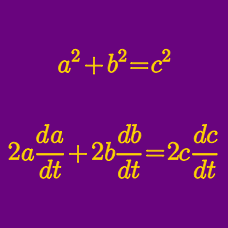Calculus

# Related Rates - 3D Geometry

The radius of a sphere increases by $1$ cm per second. If $V$ is the volume of the sphere and $t$ is time measured in seconds, what is $\frac{dV}{dt}$ (in cm/s) for $r= 13$ ?

The side length $x$ of a cube increases by $2$ cm per minute. If $V$ denotes the volume of the cube in $\text{cm}^3$, and $t$ denotes time measured in minutes, what is the change in volume over time $\frac{dV}{dt}$ (in $\text{ cm}^3/\text{min}$) for $x=6$ cm?

The radius $r$ of a sphere increases by $1$ cm per second. If $S$ denotes the surface area of the sphere, and $t$ denotes time measured in seconds, what is $\frac{dS}{dt}$ (in $\text{ cm}^2/\text{s}$) for $r= 9 \text{ cm}$?

A spherical fish tank of radius 10 meters is being filled with at a constant rate of $R\pi$ cubic meters of water per second. At height 6 meters, the rate of change of the height is 1 meter per second. What is the value of $R$?

Details and assumptions

The height is measured from the bottom of the sphere (i.e. in contact with the ground).A circular conical reservoir, vertex down, has depth $14\text{ ft}$ and top radius $7\text{ ft}.$ Water is leaking out at the rate of $\frac{1}{3}$ cubic feet per second. Find the rate at which the water level is dropping when the diameter of the surface is $7\text{ ft}.$

×